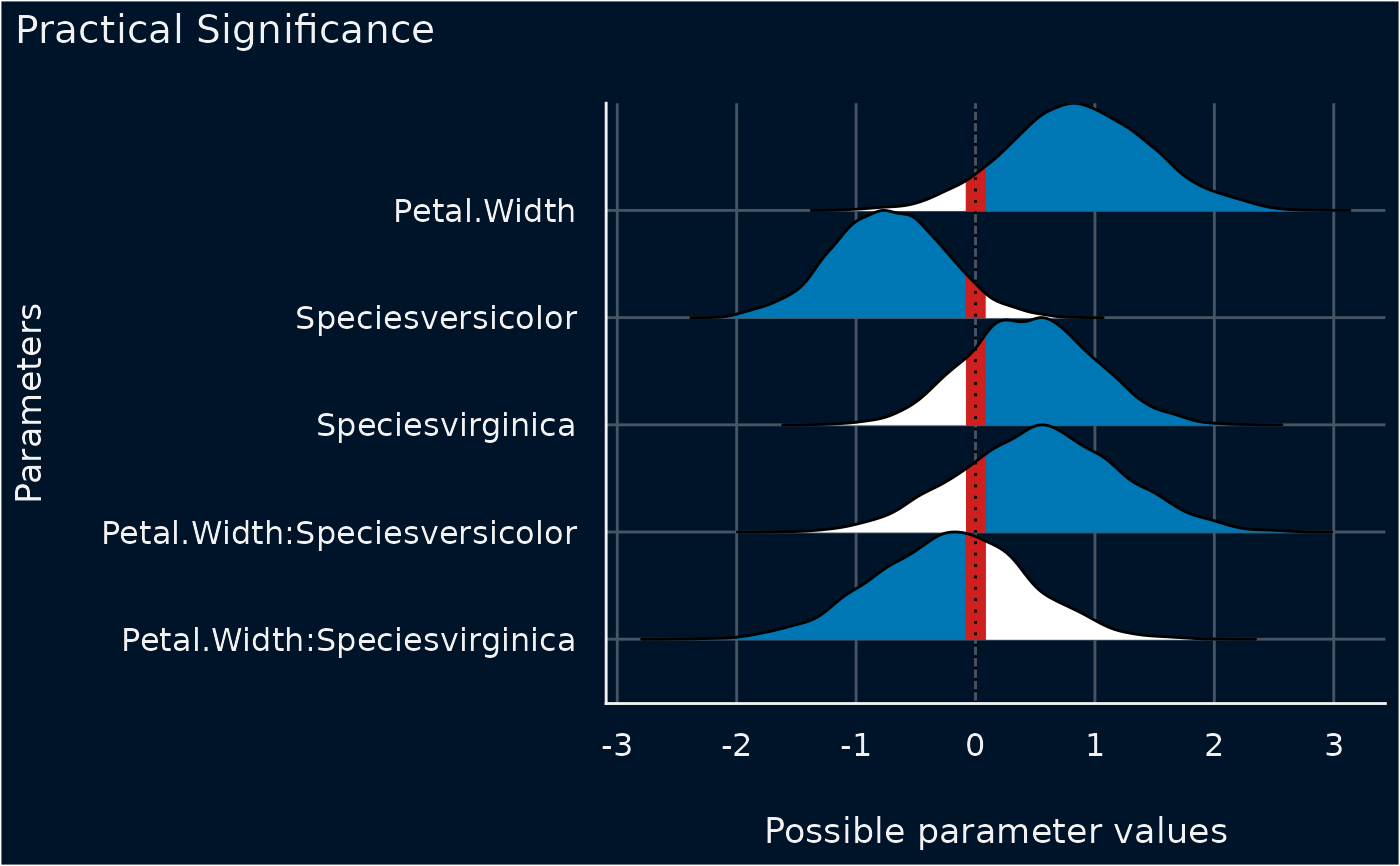The plot() method for the bayestestR::p_significance() function.

## Usage

# S3 method for see_p_significance
plot(
x,
data = NULL,
show_intercept = FALSE,
priors = FALSE,
priors_alpha = 0.4,
n_columns = 1,
...
)

## Arguments

x

An object.

data

The original data used to create this object. Can be a statistical model.

show_intercept

Logical, if TRUE, the intercept-parameter is included in the plot. By default, it is hidden because in many cases the intercept-parameter has a posterior distribution on a very different location, so density curves of posterior distributions for other parameters are hardly visible.

priors

Logical. If TRUE, prior distributions are simulated (using bayestestR::simulate_prior()) and added to the plot.

priors_alpha

Numeric value specifying alpha for the prior distributions.

n_columns

For models with multiple components (like fixed and random, count and zero-inflated), defines the number of columns for the panel-layout. If NULL, a single, integrated plot is shown.

...

Arguments passed to or from other methods.

## Value

A ggplot2-object.

## Examples

# \donttest{
if (require("bayestestR") && require("rstanarm")) {
set.seed(123)
m <<- stan_glm(Sepal.Length ~ Petal.Width * Species, data = iris, refresh = 0)
result <- p_significance(m)
plot(result)
}# }